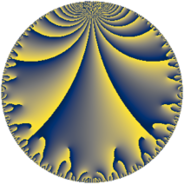# Properties

 Label 11.9.dLevel $11$ Weight $9$ Character orbit 11.d Rep. character $\chi_{11}(2,\cdot)$ Character field $\Q(\zeta_{10})$ Dimension $28$ Newform subspaces $1$ Sturm bound $9$ Trace bound $0$

# Related objects

## Defining parameters

 Level: $$N$$ $$=$$ $$11$$ Weight: $$k$$ $$=$$ $$9$$ Character orbit: $$[\chi]$$ $$=$$ 11.d (of order $$10$$ and degree $$4$$) Character conductor: $$\operatorname{cond}(\chi)$$ $$=$$ $$11$$ Character field: $$\Q(\zeta_{10})$$ Newform subspaces: $$1$$ Sturm bound: $$9$$ Trace bound: $$0$$

## Dimensions

The following table gives the dimensions of various subspaces of $$M_{9}(11, [\chi])$$.

Total New Old
Modular forms 36 36 0
Cusp forms 28 28 0
Eisenstein series 8 8 0

## Trace form

 $$28 q - 5 q^{2} + 144 q^{3} + 951 q^{4} - 708 q^{5} - 4485 q^{6} + 5470 q^{7} - 3845 q^{8} - 14225 q^{9} + O(q^{10})$$ $$28 q - 5 q^{2} + 144 q^{3} + 951 q^{4} - 708 q^{5} - 4485 q^{6} + 5470 q^{7} - 3845 q^{8} - 14225 q^{9} + 38567 q^{11} + 63954 q^{12} - 12500 q^{13} - 49262 q^{14} - 211056 q^{15} + 65335 q^{16} + 368200 q^{17} - 316350 q^{18} - 442685 q^{19} - 101488 q^{20} + 1686925 q^{22} + 704764 q^{23} - 426415 q^{24} - 960933 q^{25} + 659122 q^{26} - 1523847 q^{27} - 2014380 q^{28} - 795320 q^{29} + 680860 q^{30} + 1317554 q^{31} - 3224101 q^{33} + 897538 q^{34} + 8132260 q^{35} + 8527262 q^{36} + 8163354 q^{37} + 1456780 q^{38} - 14657890 q^{39} - 24701940 q^{40} - 13192700 q^{41} + 3806030 q^{42} - 8229340 q^{44} + 17701416 q^{45} + 38601510 q^{46} + 11954754 q^{47} + 49075784 q^{48} - 11198359 q^{49} - 41838705 q^{50} - 41019825 q^{51} - 81977530 q^{52} - 2537916 q^{53} + 40359452 q^{55} + 96646244 q^{56} + 88596375 q^{57} - 13252700 q^{58} + 49581537 q^{59} - 58912456 q^{60} - 29302910 q^{61} - 207755000 q^{62} - 178685550 q^{63} + 22221799 q^{64} + 119858070 q^{66} + 105502894 q^{67} + 335519000 q^{68} + 32103578 q^{69} + 228302300 q^{70} + 30044916 q^{71} - 375829355 q^{72} - 272936400 q^{73} - 400896650 q^{74} + 30339519 q^{75} + 23326180 q^{77} + 387475480 q^{78} + 319699810 q^{79} + 208513052 q^{80} + 403883776 q^{81} - 197581295 q^{82} - 558510965 q^{83} - 721672470 q^{84} - 360970180 q^{85} + 119645907 q^{86} + 85623835 q^{88} + 317966134 q^{89} + 1020614800 q^{90} + 285678712 q^{91} + 599604784 q^{92} - 125102972 q^{93} - 650361480 q^{94} - 641153840 q^{95} - 523100900 q^{96} + 85897149 q^{97} - 149389979 q^{99} + O(q^{100})$$

## Decomposition of $$S_{9}^{\mathrm{new}}(11, [\chi])$$ into newform subspaces

Label Dim $A$ Field CM Traces $q$-expansion
$a_{2}$ $a_{3}$ $a_{5}$ $a_{7}$
11.9.d.a $28$ $4.481$ None $$-5$$ $$144$$ $$-708$$ $$5470$$